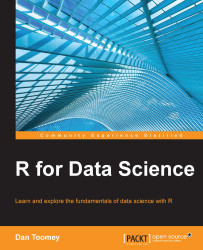•#### R for Data Science#### Overview of this book

R for Data ScienceCreditswww.PacktPub.comPrefaceFree Chapter
Data Mining PatternsData Mining SequencesText MiningData Analysis – Regression AnalysisData Analysis – CorrelationData Analysis – ClusteringData Visualization – R GraphicsData Visualization – PlottingData Visualization – 3DMachine Learning in ActionPredicting Events with Machine LearningSupervised and Unsupervised LearningIndex## Chapter 12. Supervised and Unsupervised Learning

The two basic methods of machine learning are supervised and unsupervised machine learning. In machine learning, we are usually dealing with a target variable and predictor variables. The target variable is the object of the prediction or what we are trying to learn. We want to learn how to predict that variable going forward. The predictor variables are the variables we put into our model to obtain information about the target variable. We want to learn how changes in our predictor variables affect the target variable.

Supervised learning involves the use of a target variable and a number of predictor variables that are put into a model to enable the system to predict the target. This is also known as predictive modeling.

Unsupervised modeling has no target variable. We want to discover the predictor variables that are present. This is sometimes called pattern discovery.

This chapter covers techniques in R programming for supervised and unsupervised...